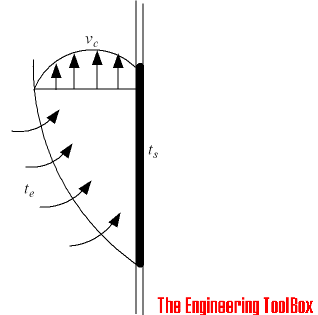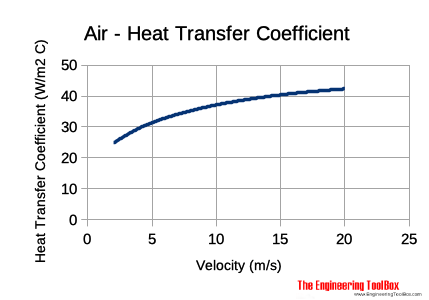Engineering ToolBox - Resources, Tools and Basic Information for Engineering and Design of Technical Applications!

# Convective Heat Transfer

## Heat transfer between a solid and a moving fluid is called convection. This is a short tutorial about convective heat transfer.

Heat energy transferred between a surface and a moving fluid with different temperatures - is known as convection.

In reality this is a combination of diffusion and bulk motion of molecules. Near the surface the fluid velocity is low, and diffusion dominates. At distance from the surface, bulk motion increases the influence and dominates.Convective heat transfer can be

• forced or assisted convection
• natural or free convection

### Forced or Assisted Convection

Forced convection occurs when a fluid flow is induced by an external force, such as a pump, fan or a mixer.

### Natural or Free Convection

Natural convection is caused by buoyancy forces due to dens ity differences caused by temperature variations in the fluid. At heating the density change in the boundary layer will cause the fluid to rise and be replaced by cooler fluid that also will heat and rise. This continues phenomena is called free or natural convection.

Boiling or condensing processes are also referred to as a convective heat transfer processes.

• The heat transfer per unit surface through convection was first described by Newton and the relation is known as the Newton's Law of Cooling.

The equation for convection can be expressed as:

q = hc A dT                                         (1)

where

q = heat transferred per unit time (W, Btu/hr)

A = heat transfer area of the surface (m2, ft2)

hc = convective heat transfer coefficient of the process (W/(m2oC, Btu/(ft2 h oF))

dT = temperature difference between the surface and the bulk fluid (oC, F)

### Heat Transfer Coefficients - Units

• 1 W/(m2K) = 0.85984 kcal/(h m2 oC) = 0.1761 Btu/(ft2 h oF)
• 1 Btu/(ft2 h oF) = 5.678 W/(m2 K) = 4.882 kcal/(h m2 oC)
• 1 kcal/(h m2 oC) = 1.163 W/(m2K) = 0.205 Btu/(ft2 h oF)

### Convective Heat Transfer Coefficients

Convective heat transfer coefficients - hc - depends on type of media, if its gas or liquid, and flow properties such as velocity, viscosity and other flow and temperature dependent properties.

Typical convective heat transfer coefficients for some common fluid flow applications:

• Free Convection - air, gases and dry vapors : 0.5 - 1000 (W/(m2K))
• Free Convection - water and liquids: 50 - 3000 (W/(m2K))
• Forced Convection - air, gases and dry vapors:  10 - 1000 (W/(m2K))
• Forced Convection - water and liquids:  50 - 10000 (W/(m2K))
• Forced Convection - liquid metals:  5000 - 40000 (W/(m2K))
• Boiling Water : 3.000 - 100.000 (W/(m2K))
• Condensing Water Vapor: 5.000 - 100.000 (W/(m2K))

#### Convective Heat Transfer Coefficient for Air

The convective heat transfer coefficient for air flow can be approximated to

hc = 10.45 - v + 10 v1/2                                     (2)

where

hc = heat transfer coefficient (kCal/m2h°C)

v = relative speed between object surface and air (m/s)

Since

1 kcal/m2h°C = 1.16 W/m2°C

- (2) can be modified to

hcW = 12.12 - 1.16 v + 11.6 v1/2                                     (2b)

where

hcWheat transfer coefficient (W/m2°C)

Note! - this is an empirical equation and can be used for velocities 2 to 20 m/s.### Example - Convective Heat Transfer

A fluid flows over a plane surface 1 m by 1 m. The surface temperature is 50oC, the fluid temperature is 20oC and the convective heat transfer coefficient is 2000 W/m2oC. The convective heat transfer between the hotter surface and the colder air can be calculated as

q = (2000 W/(m2oC)) ((1 m) (1 m)) ((50 oC) - (20 oC))

= 60000 (W)

= 60 (kW)

### Convective Heat Transfer Calculator

A - surface area (m2)

tsurface - surface temperature (oC)

tair  - air temperature (oC)

hc - convective heat transfer coefficient (W/(m2K))

Heat Transfer =   (W)

### Convective Heat Transfer Chart## Related Topics

### • Heat Loss and Insulation

Heat loss from pipes, tubes and tanks - with and without insulation - foam, fiberglass, rockwool and more.

### • Heat Loss and Insulation

Steam and condensate pipes - heat loss uninsulated and insulated pipes, insulation thickness and more.

### • Thermodynamics

Work, heat and energy systems.

## Related Documents

### Convective Air Flows from Typical Heat Sources

Convective air flows from typical heat sources like people, computers, radiators and more.

### Wind Chill Index

Effective Temperature of air in movement.

## Engineering ToolBox - SketchUp Extension - Online 3D modeling!

Add standard and customized parametric components - like flange beams, lumbers, piping, stairs and more - to your Sketchup model with the Engineering ToolBox - SketchUp Extension - enabled for use with the amazing, fun and free SketchUp Make and SketchUp Pro . Add the Engineering ToolBox extension to your SketchUp from the SketchUp Pro Sketchup Extension Warehouse!

Translate

## Privacy

We don't collect information from our users. Only emails and answers are saved in our archive. Cookies are only used in the browser to improve user experience.

Some of our calculators and applications let you save application data to your local computer. These applications will - due to browser restrictions - send data between your browser and our server. We don't save this data.